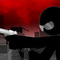...    players online request your hacks

# Sift Heads: Street Wars - Prologue HackedName:
Sift Heads: Street Wars - Prologue
Rate:
(Avg. 4.72) ...
Categories:
Shooting Stick Gamesfree.ca Click to find more games like this.
Cheat: Invulnerability.

Invulnerability.

## Game Description

In this new game series you will find the same bloody violence and story style you're used to, but with a totally new 3D environment. In this prologue of Street Wars Vinnie is confronted with all 3 organizations on his back, the Italians, the Yakuza and the Russians. Play the game and find out what happens.

## How To Play

W,A,S,D or ARROW keys to move around.
SHIFT to run.
E to interact with objects or speak to characters.
Mouse to aim and shoot.
SPACEBAR to holster weapon.
1,2,3 keys to change weapons.

Feedback for Sift Heads: Street Wars - Prologue

• collllllllllllllllllllllllllllllllllllllllllllllllllllllllllllllllllllllllllllllllllllllllllllllllllllllllllllllllllllllllllllllllllllllllllllllllllllllllllllllllllllllllllllllllllllllllllllllllllllllllllllllllllllllllll:))))))))))))))))))))))))))))))))))))))))))))))))))))))))))))))))))))))))))))))))))))))))))))))))))))))))))))))))))))))))))))))))))))))))))))))))))))))))))))))))))))))))))))))))))))))))))))))))))))))))))))))))))))))))))))))))))))))))))))))))))))))))))))))))))))))))))))))))))))))))))))))))))))))))))))))))))))))))))))))))))))))))))))))))))))))))))))))))))))))))))))))))))))))))))))))))))))))))))))))))

• i love this game is so collllllllllllllll
• anl is a jerk,gay,nerd,jerk jerk jerk jerk.

who ever scroll down is like anl
• super game . thanks hacked free games
• can you plese put infinite ammo on all weapons•